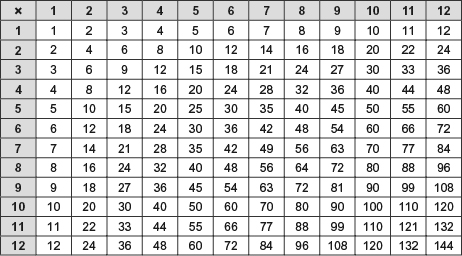Succeed with maths – Part 1

Start this free course now. Just create an account and sign in. Enrol and complete the course for a free statement of participation or digital badge if available.

Free course

# 2.1 Everyday multiplication

Just as with addition and subtraction there are a number of ways that you can carry out multiplication, in your head, on paper or using a calculator. You’ll probably find for more complicated examples you will need a calculator but if you don’t have one handy remembering how to do this on paper will be a great help. Of course, a good knowledge of the multiplication (times) tables will also be a bonus!

To make sure that you are happy with multiplying on paper have a look at a couple of examples. Suppose that you need to work out what 9 lots of £32.50 when you are out shopping.

You need to set up the calculation so that the smaller number is on the bottom, as shown below (you have to do less work then!). Working from right to left multiply each number in turn, writing the result in line with the appropriate column. If the result is greater than 9, remember you will need to carry to the next column.

If you need to multiply by a number that is 10 or more, then follow the same procedure as described above to set up the calculation. Then multiply each number in the top row by each digit in the lower number in turn, finally adding up the two results. When multiplying 3965 by 25, start with the 5 and then move onto the 2. But you are not really multiplying by 2 but by 20 (2 is in the 10s place). To take this into account, add a zero to the beginning of the 2nd line of working, as shown below.

The next activity will give you a chance to practise this. Here’s a reminder of your times tables.Figure _unit3.2.1 Figure 5 Times table

If you like you can download [Tip: hold Ctrl and click a link to open it in a new tab. (Hide tip)] and print a version of this table.

## Activity _unit3.2.1 Activity 3 Multiplication

Timing: Allow approximately 10 minutes

On a piece of paper, perform the following operations by hand.

(a) 348 × 37

### Comment

For multiplication, begin by writing the problem vertically and lining up the digits in the units place.

Thus, the answer is 12876. (This seems reasonable since 300 × 40 = 12000.)

(b) 560 × 23

Thus, the answer is 12880. (This seems reasonable since 600 × 20 = 12000.)

Now you’ve looked at multiplication, it’s time for division, a close relation of multiplication.